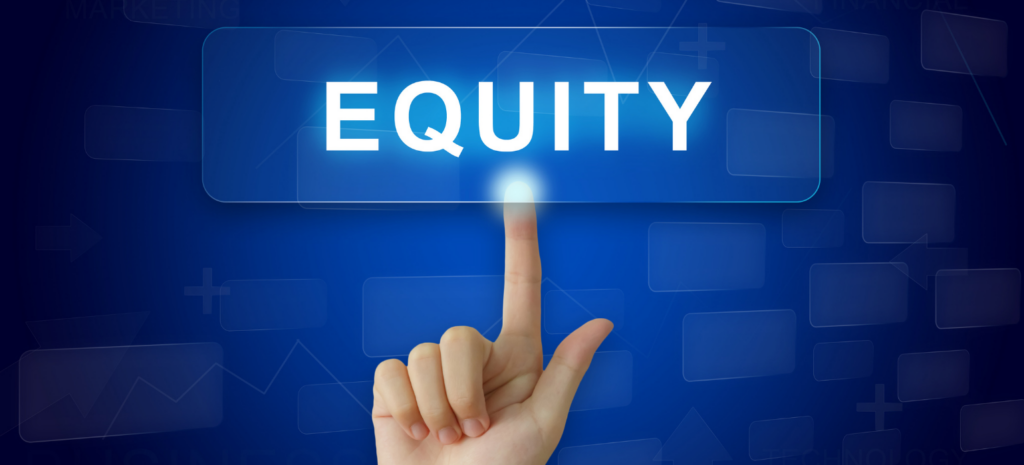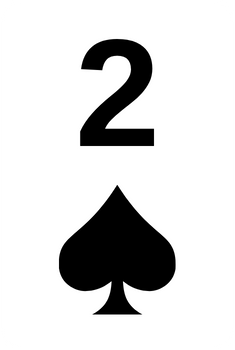## What is equity in poker?## What is equity in poker?

In this article, you will learn what equity is in poker, and how to calculate the EV (expected value) of your plays. Knowledge of equity and how your equity relates to the EV of your plays is exceptionally important to making fundamentally good winning decisions at the table.

Not knowing what is in this article would make it impossible for anyone to win at poker long term. Simply reading this article will not make you millions, but what this article will do is function as a steppingstone to future success. Keep reading to invest in yourself.

Explanation of terms

Equity is the term to describe your chances of winning the pot assuming no further betting. If you have the nuts on the river, like AA on 7J2 6 A, then your equity is 100%.

Expected value is the term to describe how much you will win or lose on average in the long run when you make a play. And just to clarify, a play can be either folding, checking, betting, or raising.

To calculate our EV, we need to know how much we will win, and how often we will win. How often we will win is our equity. We also need to know the opposite; how much we will lose, and how often we will lose.

The formula looks like this: EV = (Amount you win * How often you win) – (Amount you lose * How often you lose).
It can be rewritten like this: EV = (\$W * %W) – (\$L * %L).

Example 1
The pot is: 100.

Flop:Your hand – Flush draw with:What is your equity in this hand, and what would the EV be of calling an all-in from your opponent? Using an equity calculator (also known as an odds calculator), we easily find out that your flush draw has around 25% equity.

It is worth noting that your chance of hitting a flush by the river is greater than 25%, but sometimes, when you improve to a flush, your opponent will also have improved, but to a full house, which beats your flush.

Since we know your equity is 25%, and there is no outcome that splits this pot, we automatically know that our opponent has the inverse equity = 75%.

We can now plug this into the formula: EV = (\$W * 0.25) – (\$L * 0.75).

All we need to know now is how much money you expect to win when you win, and how you lose when you lose.

When your opponent goes all-in for 100, the pot is 100 + 100. Thus, you expect to win 200. If you call, you are risking 100 to win 200.

We can now plug this into the formula: EV = (200 * 0.25) – (100 * 0.73).

Since 200 * 0.25 = 50 and 100 * 0.75 = 75, we could easily rewrite this formula as: EV = 50 – 75.

This results in negative EV, or more specifically, -25.

We can clearly see that calling here, with our flush draw, if we know our opponent has a set is a clearly losing play, and it is even losing quite a lot.

Example 2

Let us take the same example, but without knowing our opponent’s cards. We will instead give our opponent what is referred to as a range of possible hands. This example range will include sets, two pairs, one pairs, and certain bluffs, like draws of his own.

The pot is: 100.

Flop:Your hand – Flush draw with:Using the same equity calculator, we can see that our specific hand has 43% equity against the opponent’s range.

We can now redo the calculations and see if a call would be profitable.

EV = (200 * 0.43) – (100 * 0.57).

We can simplify this to EV = 86 – 57. This results in a positive EV of 29.

As you can see from these examples, equity and expected value are linked and vital to understand for long term poker success. With that being said, none of us are equity calculators, and none of us can successfully do intricate math adjusting for various ranges at the table when we have less than 30 seconds to act. We practice theoretically off the tables and do our best practically at the tables.

Pot commitment

Sometimes, the pot is so big relative to your opponent’s bet that you cannot fold. We call this being pot committed. Of course, you always can fold to a bet, but if the pot is 100 on the turn with another card to come on the river, and your opponent bets the mere sum of 1, it would be mathematical suicide to let go of your hand.

We can prove this with the same EV formula, but I will let you do that on your own. A short disclaimer is that if you really do have 0% equity, then obviously calling any amount would be negative EV, but since we never know our opponent’s exact whole cards, we can rarely expect our equity to actually be 0%.

Fold equity

An important concept related to equity is fold equity. If you can make a bet or raise and have your opponent fold 100% of the time, then obviously, you have 100% equity in the hand. This is where bluffing comes in. You may have the nut low with 0% equity, but if your opponent folds to your bet, that does not matter.

Once again, we can prove this with the EV formula. Let us say that you have 0% equity going all-in with 100 into a pot of 100. You are in other words, risking 100 to win the 100 that is in the middle. Then let us say that your opponent is going to fold a chunky 70% of the time. In such a case, the formula would be written out like this: EV = (100 * 0.7) – (100 * 0.3). This nets a positive EV of 40. You can play around with the numbers and see for yourself, but considering fold equity is particularly important when raising a draw.

A draw is often just a high card hand with the potential to make a much better one, but if you get a draw all-in, you are going to be an underdog. Let us say that you have 33% equity with a draw, and you go all-in, but your opponent never ever folds, meaning you have 0% fold equity.

In this case, it equals out to (100 * 0.33) – (100 * 0.67) = -34.

But what if you had 20% fold equity? Meaning your opponent will actually fold to your shove 20% of the time. Well now you have 33% equity when called, as well as an added 20% equity, because your opponent folds that often. The EV would now be (100 * 0.53) – (100 * 0.47) = 6.

Equity and expected value are a bit challenging to learn as a beginner, but they are particularly important concepts that you need to fully grasp if you want to become a highly successful poker player.

Related Content

HOW?

Sign-up in the app or browser.